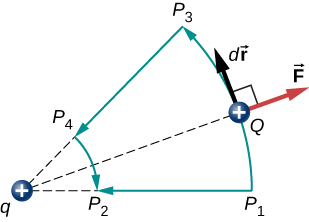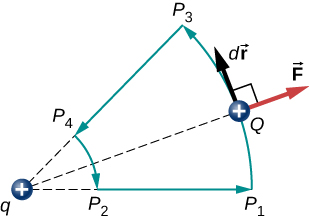# 7.1 Electric potential energy  (Page 2/5)

 Page 2 / 5

Check Your Understanding If Q has a mass of $4.00\phantom{\rule{0.2em}{0ex}}\mu \text{g},$ what is the speed of Q at ${r}_{2}?$

$K=\frac{1}{2}\phantom{\rule{0.2em}{0ex}}m{v}^{2},\phantom{\rule{0.2em}{0ex}}v=\sqrt{2\frac{K}{m}}=\sqrt{2\frac{4.5\phantom{\rule{0.2em}{0ex}}×\phantom{\rule{0.2em}{0ex}}{10}^{-7}\phantom{\rule{0.2em}{0ex}}\text{J}}{4.00\phantom{\rule{0.2em}{0ex}}×\phantom{\rule{0.2em}{0ex}}{10}^{-9}\phantom{\rule{0.2em}{0ex}}\text{kg}}}=15\phantom{\rule{0.2em}{0ex}}\text{m/s}$

In this example, the work W done to accelerate a positive charge from rest is positive and results from a loss in U , or a negative $\text{Δ}U$ . A value for U can be found at any point by taking one point as a reference and calculating the work needed to move a charge to the other point.

## Electric potential energy

Work W done to accelerate a positive charge from rest is positive and results from a loss in U , or a negative $\text{Δ}U$ . Mathematically,

$W=\text{−}\text{Δ}U.$

Gravitational potential energy and electric potential energy are quite analogous. Potential energy accounts for work done by a conservative force and gives added insight regarding energy and energy transformation without the necessity of dealing with the force directly. It is much more common, for example, to use the concept of electric potential energy than to deal with the Coulomb force directly in real-world applications.

In polar coordinates with q at the origin and Q located at r , the displacement element vector is $d\stackrel{\to }{\text{l}}=\stackrel{^}{\text{r}}\phantom{\rule{0.2em}{0ex}}dr$ and thus the work becomes

${W}_{12}=\text{−}kqQ{\int }_{{r}_{1}}^{{r}_{2}}\frac{1}{{r}^{2}}\stackrel{^}{\text{r}}·\stackrel{^}{\text{r}}dr=kqQ\frac{1}{{r}_{2}}-kqQ\frac{1}{{r}_{1}}.$

Notice that this result only depends on the endpoints and is otherwise independent of the path taken. To explore this further, compare path ${P}_{1}$ to ${P}_{2}$ with path ${P}_{1}{P}_{3}{P}_{4}{P}_{2}$ in [link] .Two paths for displacement P 1 to P 2 . The work on segments P 1 P 3 and P 4 P 2 are zero due to the electrical force being perpendicular to the displacement along these paths. Therefore, work on paths P 1 P 2 and P 1 P 3 P 4 P 2 are equal.

The segments ${P}_{1}{P}_{3}$ and ${P}_{4}{P}_{2}$ are arcs of circles centered at q . Since the force on Q points either toward or away from q , no work is done by a force balancing the electric force, because it is perpendicular to the displacement along these arcs. Therefore, the only work done is along segment ${P}_{3}{P}_{4},$ which is identical to ${P}_{1}{P}_{2}.$

One implication of this work calculation is that if we were to go around the path ${P}_{1}{P}_{3}{P}_{4}{P}_{2}{P}_{1},$ the net work would be zero ( [link] ). Recall that this is how we determine whether a force is conservative or not. Hence, because the electric force is related to the electric field by $\stackrel{\to }{\text{F}}=q\stackrel{\to }{\text{E}}$ , the electric field is itself conservative. That is,

$\oint \stackrel{\to }{\text{E}}·d\stackrel{\to }{\text{l}}=0.$

Note that Q is a constant.A closed path in an electric field. The net work around this path is zero.

Another implication is that we may define an electric potential energy. Recall that the work done by a conservative force is also expressed as the difference in the potential energy corresponding to that force. Therefore, the work ${W}_{\text{ref}}$ to bring a charge from a reference point to a point of interest may be written as

${W}_{\text{ref}}={\int }_{{r}_{\text{ref}}}^{r}\stackrel{\to }{\text{F}}·d\stackrel{\to }{\text{l}}$

and, by [link] , the difference in potential energy $\left({U}_{2}-{U}_{1}\right)$ of the test charge Q between the two points is

$\text{Δ}U=\text{−}{\int }_{{r}_{\text{ref}}}^{r}\stackrel{\to }{\text{F}}·d\stackrel{\to }{\text{l}}.$

Therefore, we can write a general expression for the potential energy of two point charges (in spherical coordinates):

$\text{Δ}U=\text{−}{\int }_{{r}_{\text{ref}}}^{r}\frac{kqQ}{{r}^{2}}dr=\text{−}{\left[-\frac{kqQ}{r}\right]}_{{r}_{\text{ref}}}^{r}=kqQ\left[\frac{1}{r}-\frac{1}{{r}_{\text{ref}}}\right].$

We may take the second term to be an arbitrary constant reference level, which serves as the zero reference:

Maxwell's stress tensor is
Yes
doris
neither vector nor scalar
Anil
if 6.0×10^13 electrons are placed on a metal sphere of charge 9.0micro Coulombs, what is the net charge on the sphere
18.51micro Coulombs
ASHOK
Is it possible to find the magnetic field of a circular loop at the centre by using ampere's law?
Is it possible to find the magnetic field of a circular loop at it's centre?
yes
Brother
The density of a gas of relative molecular mass 28 at a certain temperature is 0.90 K kgmcube.The root mean square speed of the gas molecules at that temperature is 602ms.Assuming that the rate of diffusion of a gas in inversely proportional to the square root of its density,calculate the density of
A hot liquid at 80degree Celsius is added to 600g of the same liquid originally at 10 degree Celsius. when the mixture reaches 30 degree Celsius, what will be the total mass of the liquid?
Under which topic
doris
what is electrostatics
Study of charges which are at rest
himanshu
Explain Kinematics
Two equal positive charges are repelling each other. The force on the charge on the left is 3.0 Newtons. Using your notes on Coulomb's law, and the forces acting on each of the charges, what is the force on the charge on the right?
Using the same two positive charges, the left positive charge is increased so that its charge is 4 times LARGER than the charge on the right. Using your notes on Coulomb's law and changes to the charge, once the charge is increased, what is the new force of repulsion between the two positive charges?
Nya
A mass 'm' is attached to a spring oscillates every 5 second. If the mass is increased by a 5 kg, the period increases by 3 second. Find its initial mass 'm'
a hot water tank containing 50,000g of water is heated by an electric immersion heater rated at 3kilowatt,240volt, calculate the current
what is charge
product of current and time
Jaffar
Why always amber gain electrons and fur loose electrons? Why the opposite doesn't happen?
A closely wound search coil has an area of 4cm^2,1000 turns and a resistance of 40ohm. It is connected to a ballistic galvanometer whose resistance is 24 ohm. When coil is rotated from a position parallel to uniform magnetic field to one perpendicular to field,the galvanometer indicates a charge
Using Kirchhoff's rules, when choosing your loops, can you choose a loop that doesn't have a voltage?
how was the check your understand 12.7 solved?By Richley CrapoBy Olivia D'AmbrogioBy Rohini AjayBy Brooke DelaneyBy OpenStaxBy Wey HeyBy Stephen VoronBy OpenStaxBy Madison ChristianBy Danielrosenberger